# Netting income

For fundamental equity investors, the financial statement is the launchpad for the search for value. True, quants use financial statements too. But they spend less time on what the numbers mean, than on what they are. To produce a financial statement that adequately captures the economic (not GAAP or IFRS) position of a company is no mean feet and draws upon accounting, domain knowledge, and artistry. Data scientists and machine learning engineers are more than acutely aware of the chore of data processing and cleaning. When it comes to financial statements, the cleaning isn’t about error handling and imputation; it’s about deciding which line item merits inclusion and whether or not to adjust it. Is that non-recurring charge company XYZ seems to take every quarter really non-recurring? Or is this company keeping earnings low so that it can maximize after-tax cash flow.

Why do fundamental analysts spend all this time worrying about earnings power? Because the value of a company is the discounted value of its future cash flows. Just like you can’t fire a cannon from a canoe,1 you can’t generate a good estimate of future cash flows if the base you’re using is built on shaky ground. Hence, fundamental analysts spend a lot of time trying to build really good financial statement models.

The trouble is, how do you quantify good? Presumably, a good model will help the analyst produce better forecasts of earnings, which translate into cash flows. But therein lies the rub: the skill set required to generate a good forecast (that is an accurate one), isn’t the same as the one to build a good model of a company’s economic reality. Descriptive isn’t predictive. And by data science standards, most P&L forecasts are rudimentary at best, ad hoc at worst. The fundamental analyst spends so much time trying to gather insights about company prospects and whether incremental margins will be 20% or 30%, he or she has little time to check past prediction accuracy or run model comparisons.

What if you could take away a lot of that nuance and generalize the process? What if you could just scrap all the annoying questions about non-recurring charges, FIFO vs LIFO, and below-the-line items and go straight to the forecast? Enter the neural network!

Well, not quite yet. More like enter machine learning with the end goal of using neural networks. The question is, can we use machine learning to forecast earnings with equal or better accuracy than the brute force method of building financial models that are underpinned by a good amount of individual discretion? This is not to abuse those poor equity research analysts! We were one of them at one time. Some of the best analysts also have some of the best discretion.

Our project then, is to build a machine learning model that accurately forecasts earnings. Our hypothesis is that using a neural network could produce more accurate forecasts, but we’re indifferent if other algorithms prove to be better. This project fits within our overall neural network series, but requires a bit of a detour.

Importantly, there are a several items to unpack and sort out before we can reload our suitcase and start our journey. First, how will we quantify better? Ultimately, “better” would be better than the aggregate forecast of all forecasters out there. Or even better, better than the better ones! But that poses a problem since finding such data is not always free (and our work follows the principles of reproducible research championed by other great blogs). Moreover, there is a huge sampling bias, since only a portion of analysts (the sell-side as opposed to the buy-side2) actually publish their forecasts.

Second, different macro-economic variables affect different sectors differently. And companies within each sector also perform differently due to management (gurus or crooks), geographic exposure, and a whole host of other factors. Hence, it’s not clear that there is a single model that can generalize well across all the different sectors and CEOs. Moreover, it would be beyond the scope of this blog—as long as we hold a day job at least!—to try to aggregate the data and create a model that could even tackle such a task. Maybe Google Brain will dedicate five basis points of Alphabet’s R&D to funding our research! Until then, we’ll have to stick with a toy example, which we arbitrarily choose to be Apple.

We choose Apple, not to poke Alphabet in the eye since they haven’t funded OSM yet. But because the company is so well known and actually produces stuff—like phones and computers. Well maybe not produces, but at least assembles stuff. The point is, most people have heard of Apple and so we don’t have to spend much time explaining what it does. Nor do we have to go into great detail about its financials, at least as we plan to use them. So this post won’t require the readers to know much accounting jargon.

Third, we’re not actually going to spend a lot of time on the accounting. In fact, we want to see if machine learning can learn the accounting without knowing a darn thing about accounting. Don’t worry we’re not trying to put accounting professors out of business. We actually like a few of them!

So what’s the plan? We’ll get Apple’s financial statements for the last twenty-five years or so and build some models. First, we’ll aggregate the income statement data, assign everything above net income (“the line” if you’re an equity analyst) to the features (or independent variables) and everything below (essentially net income) to the labels (or dependent variables). Let’s, we’ll look at two of major line items everyone cares about: revenue and net income.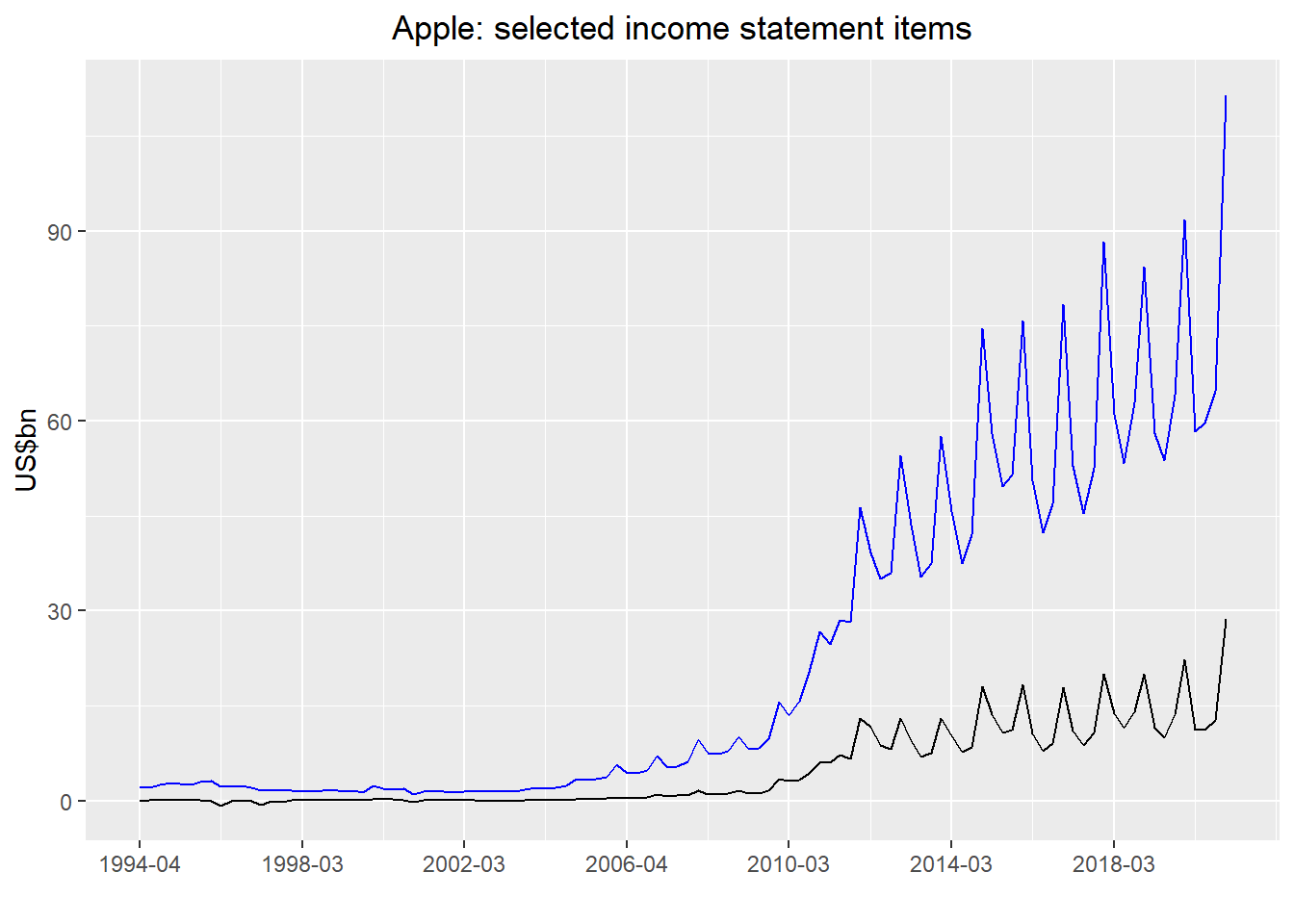While Apple’s income statement line items are pretty high level, we’ll aggregate a few of them to make the presentation and analysis straightforward. We show a bar chart of Apple’s common size income statement (all items expresses as a percent of revenue) averaged for the entire history of analysis.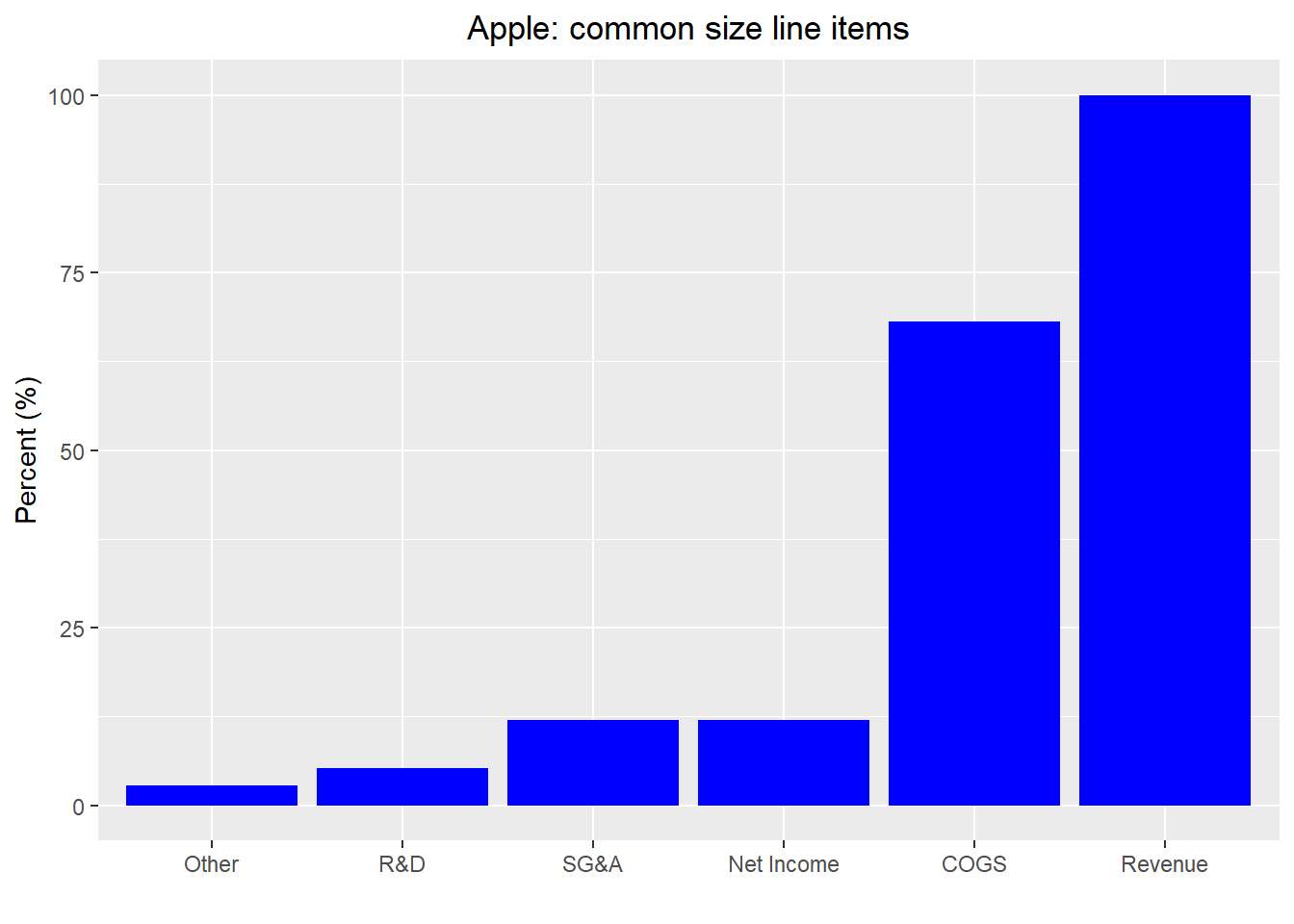Nothing remarkable to note here other than the surprising fact that R&D represents so little of revenue. Whatever the case, what happens if we run a basic linear regression model on net income vs. all other line items? Not surprisingly, the regression finds all but revenue items to have a negative size effect with revenue the only positive. The $$R^2$$ is, unsurprisingly, close to 100%. Those machines are pretty darn smart!

Forecasting the present isn’t that useful in most cases. Let’s lag the features by one quarter and see what happens. When we run the regression the $$R^2$$ turns out to be 93%. Not bad.

Sadly, when we lag the features by four quarters, we don’t see a huge improvement in explainability—the $$R^2$$ pretty much stays at 93%. While $$R^2$$ is a nice metric to use for a general sense of model fit, loss functions are usually thought to be better for gauging forecast accuracy on time series data, at least according to some stats folks.3. The intuition being that we’re trying to guess the right value rather the proportion of variance explained, so less just check how far off we are!

While the analytical toolbox of loss functions is cluttered with MSEs and RMSEs, MAEs and MAPEs, we like to use a scaled error metric. We scale the root mean squared error by the mean of the actual value to get a value, which tells us how much forecast is off relative to the average of the actual.

$$Scaled\ RMSE = \frac{\sqrt{n\sum(y_{i} - \hat{y_{i}})^{2}}}{\sum y_{i}}$$

If that makes sense to you great! Somehow seeing it in code is a little easier for us to understand. Whatever the case, when we compare the scaled RMSEs for the different lags on the training and test sets we get the following:

Table 1: Train and test split scaled RMSE
Models Train Test
One Qtr lag 55 103
Four Qtr lag 52 48

Clearly, the one-quarter lag massively overfits the data given the near doubling in the error rate on the test set. The four-quarter lag is actually much better and makes intuitive sense—for seasonal businesses like Apple’s, the prior year quarter is more likely to feature a stronger relation with period of interest than the quarter in the previous time step. Note: we’re not including the current quarter’s net income in our forecast of next quarter’s or next year’s quarterly net income. Moreover, we’re still dealing with units in dollars, which means billions. That’s going to skew results and will tend to make the explanatory power look greater than it is. Critically, while the error rate does improve in the test set, that is due to the idiosyncrasies of time series data, which we’ve seen in the past.

If we were to normalize the income statement cross-sectionally using min-max values, our $$R^2$$ would decline to 64% from 93% for the four-quarter lag model. While that might seem a lot, we’re actually more comfortable with the lower $$R^2$$ precisely because it doesn’t seem exceedingly large, potentially overfitting the data.

The trouble is when we normalize in this way it leads to odd size effects on the P&L line items, as shown in the graph below.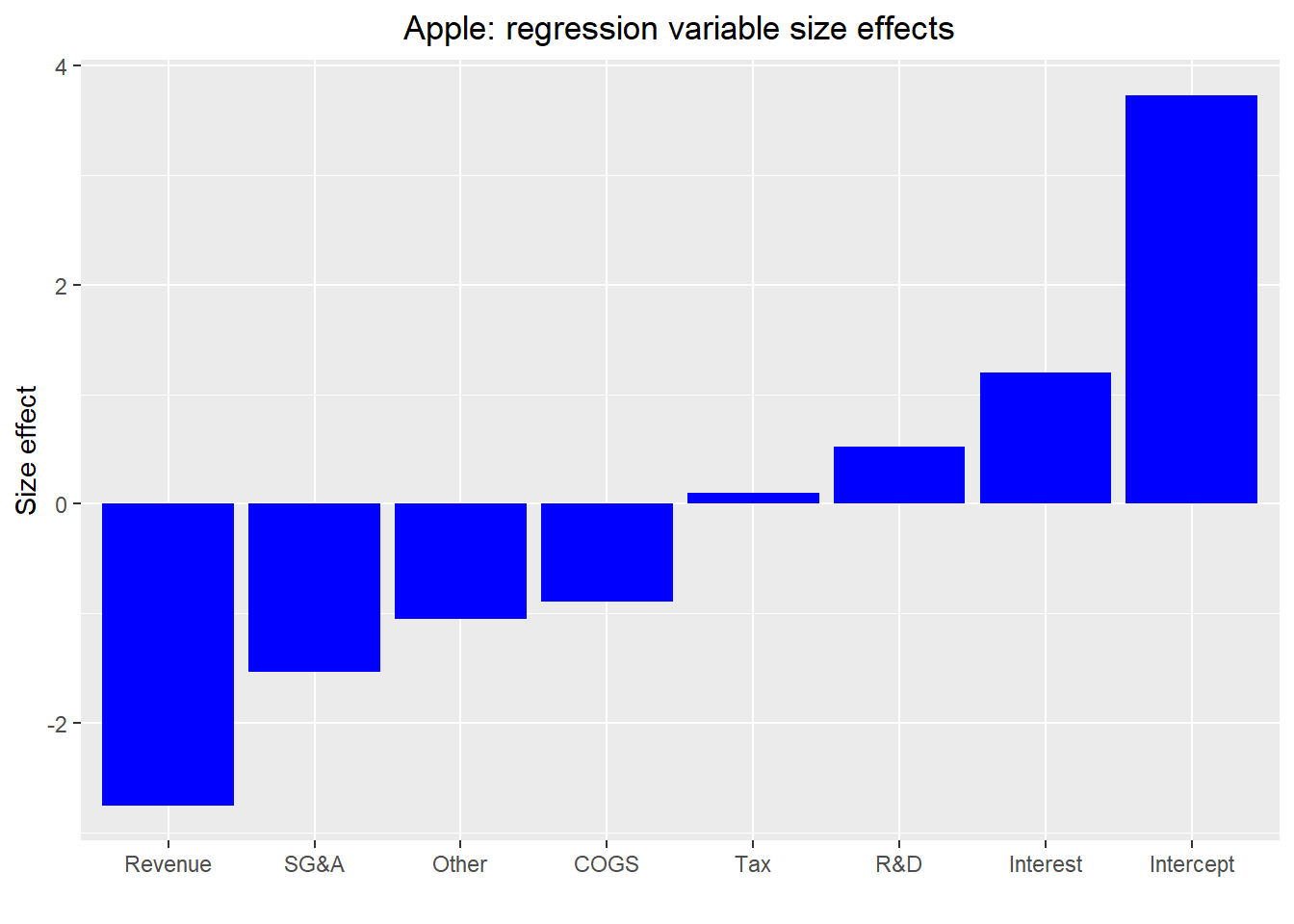Revenue has a negative size effect four quarters hence? That’s goofy. By the way, normalizing using means and standard deviations results in equally weird, but completely different size effects. So what gives? Why would we want to normalize in the first place? First, generally one doesn’t want differences in orders of magnitude to affect the feature importance, though this may not be as critical for financial statements. A big revenue number should have a bigger impact on net income than a small expense item. Second, neural networks tend to work better with normalized data; otherwise you suffer from the vanishing/exploding gradient problem, which is too large of a topic to discuss here Just know that if you don’t normalize, your error rate might not decline enough or at all.

If we transform the line items that have a negative impact on the four quarter data, the $$R^2$$ doesn’t change at 93%. And the size effects are still goofy – interest expense has a large size effect, although that result is largely random. Revenue is the only item that is statistically significant. COGS is close though. Interestingly, such significance lines up well with what most analysts who cover the stock will tell you. Gross margins are the key driver for Apple’s earnings.

If we didn’t adjust the line items for negative impacts and instead transformed them into logarithmic values, the $$R^2$$ is chopped in half to 43%. Unfortunately, we lose a lot of information since we have to exclude negative values. Nonetheless, let’s at least see what happens if we use this data transformation for a neural network.

We load the data into a neural network (NN) with two hidden layers of 100 neurons each and 500 epochs. As shown in the graph below, the NN converges with the mean-squared error (MSE) of the linear regression (the dotted horizontal line) on the same data somewhere around the 100th epoch. However, it doesn’t exactly get any better than that.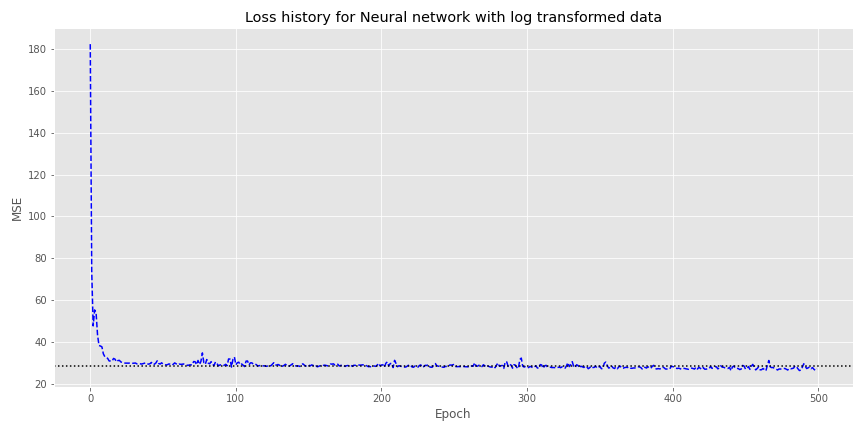Let’s go back and scale the data again. This time we’ll include the current quarter net income. As a reminder, each quarter’s line items are scaled between zero and one. Then we use this scaling to forecast the net income four quarters hence.

The linear regression using the rescaled data produces an $$R^2$$ of 80%. Not bad. How does the NN look using the same data?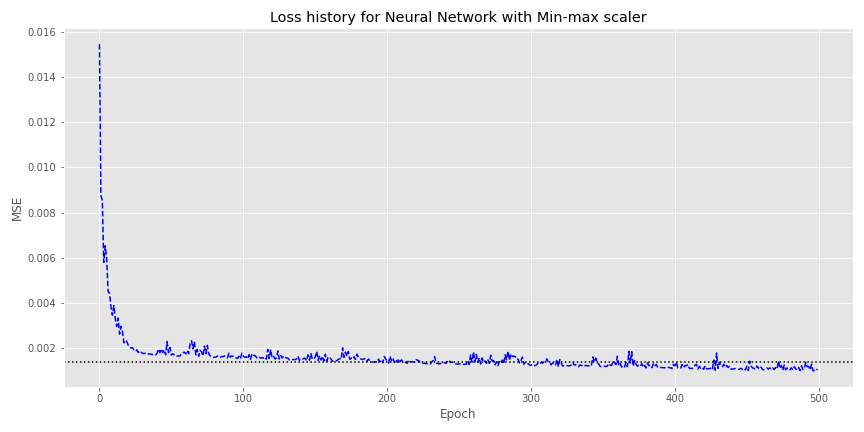Here, convergence with the linear regression MSE (the dotted horizontal line) takes a bit longer—somewhere around the 200th epoch—but the NN does see a modestly better loss around the 500th epoch compared with the log transformed model.

By now the perspicuous reader should have realized there’s something wrong with this data set up. The rescaled net income four quarters hence is based on data we wouldn’t see. That is, the model would forecast a scaled net income number of say 0.05 or 0.10. But those numbers are based on the line items in that quarter, which we won’t have until the earnings release. We’ll need to rescale with the four quarter ahead net income already in the data series.

Running the regression on that new transformation yields an $$R^2$$ of 61%. Now we’ll run the neural network and show the loss graph below.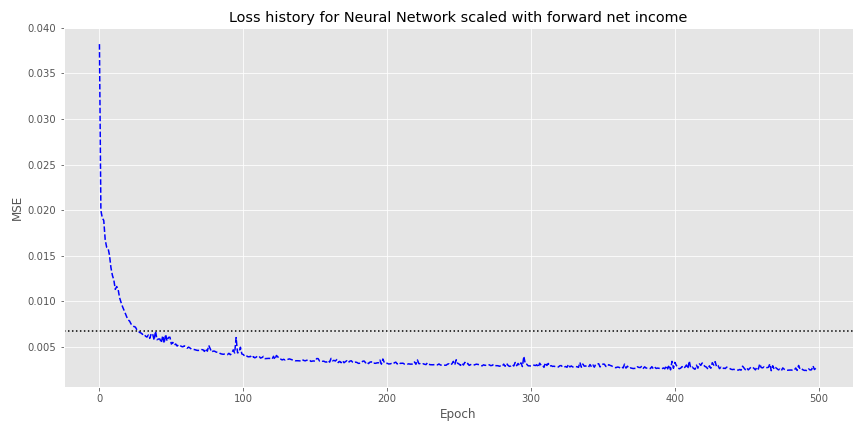The NN converges with the linear regression MSE in about 40 epochs and then continues to improve from there. Nice!

Our next steps will be to compare this to a decision tree and then test against real analyst estimates. That is, if we can find the data! If you have any questions, email us at the address below. Until then, here’s the code.

R code. Built using R 4.0.3

## Load libraries
suppressPackageStartupMessages({
library(tidyverse)
library(tidyquant)
library(reticulate)
})

# See Python code below for how we wrangled the data using the FundamentalAnalysis package
# Also all the neural network model building is found in the Python section. Once we get Keras and TensorFlow working in our R environment, we'll include that code too.

aapl <- aapl[nrow(aapl):1,]

## Graph revenue and net income
aapl %>%
ggplot(aes(x = 1:108)) +
geom_line(aes(y = revenue/1e9, color="Revenue")) +
geom_line(aes(y=netIncome/1e9, color= "Net Income")) +
scale_color_manual("", breaks = c("Revenue", "Net Income"), values = c("blue", "black"))+
xlab("") +
ylab("US$bn") + ggtitle("Apple: selected income statement items")+ scale_x_continuous(breaks = seq(1,108,16), labels = aapl$index[seq(1,108,16)]) +
theme(legend.position = "inside", plot.title=element_text(hjust=0.5))

## Graph common size income statement
aapl %>%
mutate(all_other = interestExpense + Other + incomeTaxExpense) %>%
summarize_all(function(x) mean(x/aapl$revenue)*100) %>% gather(key, value) %>% ggplot(aes(reorder(key,value),value)) + scale_x_discrete(labels= c("Other", "R&D", 'SG&A', 'Net Income', 'COGS', 'Revenue')) + geom_bar(stat='identity', fill="blue") + labs(x = "", y = "Percent (%)", title = "Apple: common size line items") + theme(plot.title = element_text(hjust=0.5)) ## Create train/test splits split <- as.integer(nrow(aapl)*.7) df <- aapl[,2:9] df_train <- df[1:split,] df_test <- df[(split+1):nrow(df),] ## Lag data df_train_lag <- df_train %>% mutate(netIncome = lead(netIncome)) df_test_lag <- df_test %>% mutate(netIncome = lead(netIncome)) ## Create first linear model mod_1 <- lm(netIncome ~., data = df_train_lag) rsq_1 <- round(broom::glance(mod_1)$r.squared, 2)*100

## Create second linear model
df_train_lag_4 <- df_train %>%

df_test_lag_4 <- df_test %>%

mod_2 <- lm(netIncome ~., data = df_train_lag_4)

rsq_2 <- round(broom::glance(mod_2)$r.squared,2)*100 ## Run predictions and RMSEs pred_train <- predict(mod_1, df_train_lag) pred_test <- predict(mod_1, df_test_lag) rmse_train <- round(sqrt(mean((pred_train - df_train_lag$netIncome)^2, na.rm = TRUE))/
mean(df_train_lag$netIncome, na.rm = TRUE),2)*100 rmse_test <- round(sqrt(mean((pred_test - df_test_lag$netIncome)^2, na.rm = TRUE))/
mean(df_test_lag$netIncome, na.rm = TRUE),2)*100 pred_train_4 <- predict(mod_2, df_train_lag_4) pred_test_4 <- predict(mod_2, df_test_lag_4) rmse_train_4 <- round(sqrt(mean((pred_train_4 - df_train_lag_4$netIncome)^2, na.rm = TRUE))/
mean(df_train_lag_4$netIncome, na.rm = TRUE),2)*100 rmse_test_4 <- round(sqrt(mean((pred_test_4 - df_test_lag_4$netIncome)^2, na.rm=TRUE))/
mean(df_test_lag_4$netIncome, na.rm = TRUE),2)*100 ## Print table of Scaled RMSE data.frame("Models"= c('One Qtr lag', 'Four Qtr lag'), "Train" = c(rmse_train, rmse_train_4), "Test" = c(rmse_test, rmse_test_4), check.names=FALSE) %>% knitr::kable(caption = "Train and test split scaled RMSE") ## Create new data frame and Min-max normalize min_max_scaler <- function(x, ...){ (x - min(x))/(max(x) - min(x)) } dat_norm <- matrix(nrow = nrow(df_train_lag_4), ncol = ncol(df_train_lag_4)) for(i in 1:nrow(dat_norm)){ dat_norm[i,] <- min_max_scaler(as.numeric(df_train_lag_4[i,])) } dat_norm <- data.frame(dat_norm) colnames(dat_norm) <- c(colnames(df_train_lag_4)[1:7], 'net_income') ## Run linear model on scaled data lm_norm <- lm(net_income ~., data = dat_norm) rsq_norm <- round(broom::glance(lm_norm)$r.squared,2)*100

## Graph size effects of scaled model
broom::tidy(lm_norm) %>%
select(term, estimate) %>%
arrange(estimate) %>%
ggplot(aes(fct_reorder(term, estimate), estimate)) +
geom_bar(stat = 'identity', position = 'dodge', fill='blue') +
scale_x_discrete(labels= c('Revenue', 'SG&A', "Other", 'COGS', "Tax", "R&D", "Interest", "Intercept")) +
geom_bar(stat='identity', fill="blue") +
labs(x = "", y = "Size effect", title = "Apple: regression variable size effects") +
theme(plot.title = element_text(hjust=0.5))

## Create data and model on line items adjusted for negative impact
df_neg <- df_train_lag_4
df_neg[, colnames(df_neg)[c(2:5,7)]] <- df_neg[, colnames(df_neg)[c(2:5,7)]]*-1

lm_neg <- lm(netIncome ~. ,data = df_neg)

rsq_neg <- round(broom::glance(lm_neg)$r.squared,2)*100 ## Create datafraem and log-transformed model log_transform <- function(x,...){ ifelse(is.finite(log10(x)),log10(x), 0) } # Note will get warnings: NaNs produced. Wish I could remove that dat_log <- apply(df_train,2,log_transform) dat_log <- data.frame(dat_log) dat_log_4 <- dat_log %>% mutate(net_inc_4 =lead(netIncome,4)) lm_log <- lm(net_inc_4 ~., data = dat_log_4) rsq_log <- round(broom::glance(lm_log)$r.squared,2)*100

## Create scaled data frame and model using current net income.
dat_mm <- matrix(nrow = nrow(df_train), ncol = ncol(df_train))
for(i in 1:nrow(dat_mm)){
dat_mm[i,] <- min_max_scaler(as.numeric(df_train[i,]))
}

dat_mm <- data.frame(dat_mm)
colnames(dat_mm) <- colnames(df_train)

dat_mm_lag  <- dat_mm %>%

lm_mm <- lm(net_inc_4 ~., data = dat_mm_lag[1:(nrow(dat_mm_lag)-4),])
rsq_mm <- round(broom::glance(lm_mm)$r.squared,2)*100 ## Create model with forward net income scaled to historical results df_train_mm <- df_train %>% mutate(net_inc_for = lead(netIncome,4)) dat_norm_1 <- matrix(nrow = nrow(df_train_mm), ncol = ncol(df_train_mm)) for(i in 1:nrow(dat_norm)){ dat_norm_1[i,] <- min_max_scaler(as.numeric(df_train_mm[i,])) } dat_norm_1 <- data.frame(dat_norm_1) colnames(dat_norm_1) <- c(colnames(df_train_mm)) lm_norm_1 <- lm(net_inc_for ~., data = dat_norm_1) rsq_norm_1 <- round(broom::glance(lm_norm_1)$r.squared,2)*100

Python code. Built using Python 3.8.3

## Load packages
import numpy as np
import pandas as pd
import matplotlib.pyplot as plt
import tensorflow as tf
from tensorflow import keras

%matplotlib inline

plt.style.use('ggplot')
plt.rcParams['figure.figsize'] = (12,6)

## For graphs
# We save the matplotlib graphs to png and import them into the blog as reticulate seems to have trouble with our graphing methods.

import os
DIR = "C:/Users/user/folder/for/images"

def save_fig_blog(fig_id, tight_layout=True, fig_extension="png", resolution=300):
path = os.path.join(DIR, fig_id + "." + fig_extension)
print("Saving figure", fig_id)
if tight_layout:
plt.tight_layout()
plt.savefig(path, format=fig_extension, dip=resolution)

try:
except FileNotFoundError:
import FundamentalAnalsysis as FA
ticker = 'AAPL'
api_key = 'your_api_key'
aapl = fa.income_statement(ticker, api_key, period="quarter")

line_items = ['revenue','costOfRevenue', 'grossProfit', 'researchAndDevelopmentExpenses', 'generalAndAdministrativeExpenses',
'sellingAndMarketingExpenses', 'otherExpenses', 'operatingExpenses',
'costAndExpenses', 'interestExpense', 'depreciationAndAmortization',
'operatingIncome', 'totalOtherIncomeExpensesNet', 'incomeBeforeTax',
'incomeTaxExpense', 'netIncome','eps', 'epsdiluted', 'weightedAverageShsOut',
'weightedAverageShsOutDil']

test = aapl.loc[line_items, '2020-12':'1994-03'].T

# After lots of testing to see which line items to include, we came up with the following. Email us if you want that part of the code. It's not pretty!
test['Other'] = test['operatingIncome'] - test['interestExpense'] - test['incomeBeforeTax']
features = ['revenue','costOfRevenue', 'researchAndDevelopmentExpenses', 'generalAndAdministrativeExpenses', 'interestExpense','Other','incomeTaxExpense']

# To CSV for R
aapl_csv = test[features+['netIncome']].reset_index()
aapl_csv.to_csv(r'C:\Users\user\folder\to\save\aapl.csv', index=None)

## Graph revenue and net income
df = aapl_csv.set_index('index').iloc[::-1]
fig, ax = plt.subplots()
ax.plot(df.index, df['revenue']/1e9, 'b-')
ax.plot(df.index, df['netIncome']/1e9, 'k-')
ax.set_ylabel('US\$bn')
ax.set_title('Apple: Selected income statement items 1994-2020')
ax.legend(['Revenue', 'Net Income'])
ax.set_xticks(range(0, len(df), 8))
plt.show()

## Graph common size income statement
aapl_csv['all_other'] = aapl_csv.loc[:, ['interestExpense', 'Other', 'incomeTaxExpense']].sum(axis=1)
ax = aapl_csv.iloc[:,[1,2,3,4,8,9]].apply(lambda x : np.mean(x/aapl_csv['revenue'])*100).sort_values().plot(kind='bar', color='blue')
ax.set_ylabel("Percent(%)")
# ax.set_xticklabels(aapl_csv.columns[[1,2,3,4,8]],rotation=0)
ax.set_xticklabels(labels = ['Other','R&D', 'SG&A', 'Net Income', 'COGS', 'Revenue'],rotation=0)
plt.show()

## Create train/test splits
# Create X and y fames
X = aapl_csv[features]
y = aapl_csv['netIncome']
X = X.iloc[::-1]
y = y.iloc[::-1]

split = int(len(X)*.7)
X_train, y_train = X[:split], y[:split]
X_test, y_test = X[split:], y[split:]

## Create first linear model
from sklearn.linear_model import LinearRegression

lr = LinearRegression()
lr.fit(X_train.values, y_train.values)

lr.score(X_train.values, y_train.values)

## Linear model with lag values
X_lag = X_train.values[:-1].astype(float)
y_for = y_train.values[1:].astype(float)

lr_1 = LinearRegression()
lr_1.fit(X_lag, y_for)
lr_1.score(X_lag, y_for)

## Create second linear model
X_lag_4 = X_train.values[:-4].astype(float)
y_for_4 = y_train.values[4:].astype(float)

lr_4 = LinearRegression()
lr_4.fit(X_lag_4, y_for_4)
lr_4.score(X_lag_4, y_for_4)

## Run predictions and RMSEs
pred_train = lr_1.predict(X_lag)
pred_test = lr_1.predict(X_test[:-1])

rmse_train = np.round(np.sqrt(np.mean((pred_train - y_for)**2))/np.mean(y_for),2)*100
rmse_test = np.round(np.sqrt(np.mean((pred_test - y_test[1:])**2))/
np.mean(y_test[1:]),2)*100

pred_train_4 = lr_4.predict(X_lag_4)
pred_test_4 = lr_4.predict(X_test[:-4])

rmse_train_4 = np.round(np.sqrt(np.mean((pred_train_4 - y_for_4)**2))/np.mean(y_for_4),2)*100
rmse_test_4 = np.round(np.sqrt(np.mean((pred_test_4 - y_test[4:])**2))/
np.mean(y_test[4:]),2)*100

## Print table of Scaled RMSE
pd.DataFrame(np.c_[[rmse_train, rmse_train_4],[rmse_test, rmse_test_4]],
index = ['One qtr lag', 'Four qtr lag'],
columns = ['Train','Test'])

## Create new data frame and Min-max normalize
dat = np.c_[X_lag_4, y_for_4]
dat_norm = np.apply_along_axis(lambda x: (x - np.min(x))/(np.max(x) - np.min(x)), 1, dat)

## Run linear model on scaled data
X_lr_n = dat_norm[:,:-1]
y_lr_n = dat_norm[:,-1]

lr_n = LinearRegression()
lr_n.fit(X_lr_n, y_lr_n)
lr_n.score(X_lr_n, y_lr_n)

X_t = np.apply_along_axis(lambda x: (x - np.min(x))/(np.max(x) - np.min(x)),1,X_lag_4)

lr_test = LinearRegression()
lr_test.fit(X_t, y_for_4)
lr_test.score(X_t, y_for_4)

## Graph size effects of scaled model
# Only did this in R.

## Create data and model on line items adjusted for negative impact
X_new = X.copy()

split = int(len(X)*.7)
X_train_new = X_new[:split]
X_test_new = X_new[split:]

lr_neg = LinearRegression()
lr_neg.fit(X_train_new.values, y_train.values)

lr_neg.score(X_train_new.values, y_train.values)

X_new_lag_4 = X_train_new.values[:-4].astype(float)

lr_4 = LinearRegression()
lr_4.fit(X_new_lag_4, y_for_4)
lr_4.score(X_new_lag_4, y_for_4)

## Create datafraem and log-transformed model
def log_transform(series):
return np.array([np.log(x) if x > 0 else 0 for x in series])

X_log = X_train.apply(lambda x: log_transform(x)).values[:-4]
y_log = log_transform(y_for_4)

lr_log = LinearRegression()
lr_log.fit(X_log, y_log)
lr_log.score(X_log, y_log)

# Create predictions and calculate MSE
pred_log = lr_log.predict(X_log)
mse_log = np.mean((pred_log - y_log)**2)
mse_log

## Create and train neural network
keras.backend.clear_session()
np.random.seed(42)
tf.random.set_seed(42)

X_log = X_train.apply(lambda x: log_transform(x)).values[:-4]
y_log = log_transform(y_for_4)

model = keras.models.Sequential([
keras.layers.Dense(100, activation = 'relu', input_shape=X_log.shape[1:]),
keras.layers.Dense(100, activation = 'relu'),
keras.layers.Dense(1)
])

history = model.fit(X_log, y_log, epochs=500)

def loss_plot(history, mse_lin, plot_title = None, save_fig=False, fig_title=None):
nn_df = pd.DataFrame(history.history)
fig, ax = plt.subplots()
ax.plot(nn_df.index, nn_df['loss'], 'b--')
ax.axhline(y=mse_lin, xmin=0, xmax=len(nn_df), color='black', ls=":")
ax.set_ylabel('MSE')
ax.set_xlabel('Epoch')
if plot_title is not None:
ax.set_title(plot_title)
if save_fig:
save_fig_blog(fig_id=fig_title)
plt.show()

loss_plot(history, mse_log, plot_title = 'Error history of log transformed Neural network', save_fig=True, fig_title='nn_1_tf_5')

## Create scaled data frame and model using current net income.
new_train = np.c_[X_train, y_train]
new_train_mm = np.apply_along_axis(lambda x: (x - np.min(x))/(np.max(x) - np.min(x)),1,new_train)
X_mm = new_train_mm[:-4,:]
y_mm = new_train_mm[4:, -1]
X_mm.shape, y_mm.shape

lin_reg_mm = LinearRegression()
lin_reg_mm.fit(X_mm, y_mm)
lin_reg_mm.score(X_mm, y_mm)

# Create predictions and calculate MSE
from sklearn.metrics import mean_squared_error
pred_mm = lin_reg_mm.predict(X_mm)
mse_mm = mean_squared_error(y_mm, pred_mm)
mse_mm

## Create and train neural network
keras.backend.clear_session()
np.random.seed(42)
tf.random.set_seed(42)

model = keras.models.Sequential([
keras.layers.Dense(100, activation='relu', input_shape=X_new.shape[1:]),
keras.layers.Dense(50, activation='relu'),
keras.layers.Dense(1)
])

history = model.fit(X_mm.astype(float), y_mm.astype(float), epochs=500)

loss_plot(history, mse_mm, plot_title='Loss history for Neural Network with Min-max scaler', save_fig=True, fig_title='nn_2_tf_5')

## Create model with forward net income scaled to historical results
new_dat = np.c_[X_train[:-4], y_train[:-4], y_for_4]
new_dat_mm = np.apply_along_axis(lambda x: (x - np.min(x))/(np.max(x) - np.min(x)),1,new_dat)

X_new_dat = new_dat_mm[:,:-1]
y_new_dat = new_dat_mm[:,-1]

lin_reg_new = LinearRegression()
lin_reg_new.fit(X_new_dat, y_new_dat)
lin_reg_new.score(X_new_dat, y_new_dat)

pred_new_dat = lin_reg_new.predict(X_new_dat)
mse_new = mean_squared_error(pred_new_dat, y_new_dat)
mse_new

keras.backend.clear_session()
np.random.seed(42)
tf.random.set_seed(42)

model = keras.models.Sequential([
keras.layers.Dense(100, activation='relu', input_shape=X_new.shape[1:]),
keras.layers.Dense(50, activation='relu'),
keras.layers.Dense(1)
])

# opt = keras.optimizers.SGD(learning_rate=0.01, momentum=0.9,clipnorm=0.95)

loss_plot(history, mse_new, plot_title='Loss history for Neural Network scaled with forward net income', save_fig=True, fig_title='nn_3_tf_5')